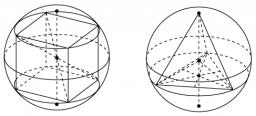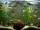# Pebble

The aquarium with internal dimensions of the bottom 40 cm × 35 cm and a height of 30 cm is filled with two-thirds of water. Calculate how many millimeters the water level in the aquarium rises by dipping a pebble-shaped sphere with a diameter of 18 cm.

x =  21.8116 mm

### Step-by-step explanation:Did you find an error or inaccuracy? Feel free to write us. Thank you!Math student
where did we get the small d?Dr Math
small d = height of water level rise. c is occupied yet, thus the next variable is d.Tips to related online calculators
Do you know the volume and unit volume, and want to convert volume units?

#### You need to know the following knowledge to solve this word math problem:

We encourage you to watch this tutorial video on this math problem:

## Related math problems and questions:

• Aquarium volumeThe aquarium has a cuboid shape and dimensions a = 0.3 m, b = 0.85 m, c =? , V = ?. What volume has a body, if after dipping into the aquarium water level rises by 28 mm?
• AquariumThe box-shaped aquarium is 40 cm high; the bottom has dimensions of 70 cm and 50 cm. Simon wanted to create an exciting environment for the fish, so he fixed three pillars to the bottom. They all have the shape of a cuboid with a square base. The base edg
• AquariumThere is 15 liters of water in a block-shaped aquarium with internal dimensions of the bottom of 25 cm and 30 cm. Find the volume of water-wetted surfaces. Express the result in dm square.
• Aquarium 6How high is the water level in the aquarium with a rectangular base 40cm and 50cm if it is filled 0,65hl of water?
• Water in aquariumThe aquarium cuboid shape with a length of 25 cm and a width of 30 cm is 9 liters of water. Calculate the areas which are wetted with water.
• SnailsHow many liters of water will fit in an aquarium with bottom dimensions of 30 cm and 25 cm and a height of 60 cm, if we pour water up to a height of 58 cm? How many most snails can we keep in an aquarium, if we know that snails need 600 cm ^ 3 of water fo
• Water levelThe glass container has a cuboid shape with dimensions of the bottom 24 cm and 12 cm. The height of the water is 26 cm. Calculate the body's volume, which is sunk into the water if the water level rise by 3 cm.
• Cuboid aquariumCuboid 25 times 30 cm. How long is third side if cuboid contains 30 liters of water?
• Aquarium heightHow high does the water in the aquarium reach, if there are 36 liters of water in it? The length of the aquarium is 60 cm and the width is 4 dm.
• Cube-shaped containerThe cube-shaped container has a height of 52 cm and a square base. The container was filled to the brim with water, then we immersed a metal cube in it, which caused 2.7 l of water to flow out of the container. After removing the cube from the water, the
• Uboid volumeCalculate the cuboid volume if the walls are 30cm², 35cm², 42cm²
• The poolThe cube-shaped pool has 140 cubic meters of water. Determine the dimensions of the bottom if the depth of the water is 200 cm and one dimension of the base is 3 m greater than the other. What are the dimensions of the pool bottom?
• HectolitersHow deep is the pool if there are 2025 hectoliters of water and the bottom dimensions are a = 15 meters b = 7.5 meters and the water level is up to 9/10 (nine-tenths) of height?
• Water tankWhat is the height of the cuboid-shaped tank with the bottom dimensions of 80 cm and 50 cm if the 480 liters of water reach 10 cm below the top?
• TrayWhat height reach water level in the tray shaped a cuboid, if it is 420 liters of water and bottom dimensions are 120 cm and 70 cm.
• AquariumCan 30 liters of water fit in a cuboid aquarium with dimensions a = 3dm b = 6dm c = 5dm?
• PrismThe volume of tetrahedral prism is 2.43 m3. Base of prism is a parallelogram in which a side 2,5dm and height ha = 18cm. Calculate the height of the prism.